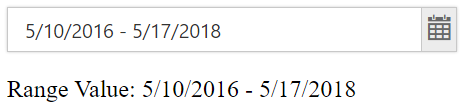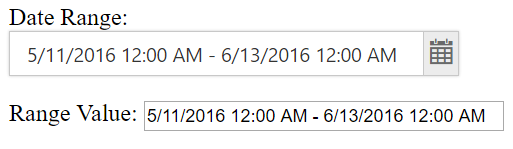# One-Way Binding

In daterange picker , one-way binding can be achieved by using “e-“ prefix with the property name . Here, One-way binding is explained with value of the daterangepicker. once the date range value has been changed and the element is focused out, the value will be below the DateRangePicker. Please check the below example code for one-way binding.

• html
• ``````<div ng-controller="dateRangeCtrl" >
<input type="text" id="daterange" ej-daterangepicker e-value="value" e-width="300px" />

<p>Range Value:</p>

</div>``````
• javascript
• ``````<script>
angular.module('syncApp', ['ejangular'])
.controller('dateRangeCtrl', function (\$scope) {
\$scope.value = "5/10/2016 - 5/17/2018";
});
</script>``````

Run the above code to get the below output.Here, once the value of the date range picker is changed and the element is focused out , the value will be updated below the daterangepicker component.

# Two-Way Binding

Two-Way binding in Angular in daterangepicker is achieved by using (ng-model) attribute. Here, once the date range value has been changed and the element is focused out, the value will be updated in the input textbox below the DateRangePicker and vice versa. Please check the below example code for two-way binding.

• html
• ``````<div ng-controller="dateRangeCtrl" >
<input type="text" id="daterange" ej-daterangepicker e-value="value" e-width="300px" />

<p>Range Value:<input ng-model="value" size="35" /></p>

</div>``````
• javascript
• ``````<script>
angular.module('syncApp', ['ejangular'])
.controller('dateRangeCtrl', function (\$scope) {
\$scope.value = "5/28/2016 12:00 AM - 5/28/2018 12:00 AM";
\$scope.enable = "true";
});
</script>``````

Run the above code to get the below output.